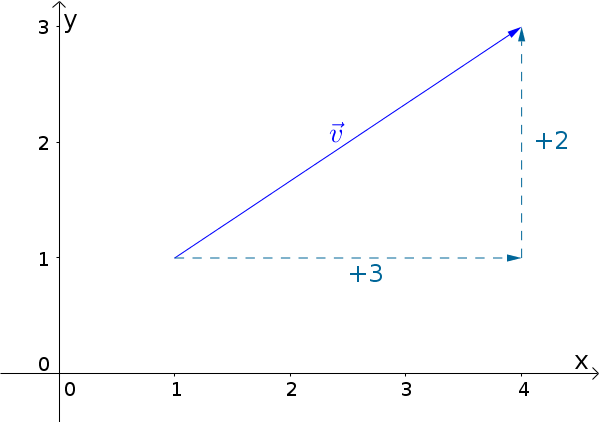Math Vectors Coordinates of a vector

# Coordinates of a vector

Vectors exist in a plane as well as in three-dimensional space. To easily represent them in the coordinate system, use the column vectors.

i

### Info

Alongside column vectors row vectors exist, as well. These are usually not important at school.

## Vectors in the plane

A vector in a plane looks as follows:

$\vec{v}=\begin{pmatrix}v_1\\v_2\end{pmatrix}$

The coordinates $v_1, v_2$ of the vector $\vec{v}$ show the shift along the coordinate axes.

### ExampleThe illustrated vector $\vec{v}=\begin{pmatrix}\color{green}{3}\\\color{red}{2}\end{pmatrix}$ has a shift by $\color{green}{+3}$ in the x-direction and $\color{red}{+2}$ in the y-direction

## Vectors in space

Vectors are also available in three-dimensional space. Logically they have one more coordinate.

$\vec{v}=\begin{pmatrix}v_1\\v_2\\v_3\end{pmatrix}$